Courses

CSS 51 Computing Laboratory (click for details)
Course Outcome(CO) & Programme Outcome(PO)
CO & PO
List of Experiments
Assignments on expression evaluation

Assignment-1

Write the pseudocode and draw a flowchart:

1. To find the sum of 5 numbers
2. To compare between two numbers and print the maximum number.
3. To print “Hello good morning”.
4. To take the marks of 5 subjects of a student. Compute the total and Average. If the average is less than 40, display FAIL', otherwise display 'PASS". Then check if the average is less than 50, display D' otherwise, check again. If the average is less than 60, then display C' otherwise, check again. If the average is less than 70, display B otherwise, check again. If the average is less than 80, display 'A' otherwise, display ‘EX’.
Implementation of conditional branching (if, if-else, switch case)

Assignment-2

1. WAP in C to swap two numbers with/without using a third variable.
2. Write a C- program to generate the following series using if else. 0 1 1 2 3 5 8 13
3. WAP to print a number is Even or Odd using if else.
4. WAP to find out biggest among 3 numbers taken from keyboard using if else.
5. WAP a program to take a character from keyboard and check whether it is vowel or not. If the character taken from keyboard is small, then display its corresponding capital value and vice versa.
Implementation of iterations (for, do while, while)

Assignment-3

1. WAP to find out the max number in an array of 10 integers.
2. WAP to take an array of 5 integers from keyboard and print the sorted array i descending order.
3. WAP to take an array of 10 floats and print reverse of the array.
4. WAP to take the marks 5 subjects of a student in an array. Compute the total. If the total is more than 40 and display the student has Passed otherwise display fail.
5. WAP to take enter 20 numbers from keyboard to an array. The number to be searched is also entered from the keyboard. Find out if the entered number is present in the array or not. If it is present, display the number of times it appears in the aray.
6. WAP to enter values in two separate 6X6 matrix find add and difference (minus) of two 6X6 matrices.
7. WAP to enter values in two separate 4X4 matrix and find multiplication of the two 4X4 matrices.
8. WAP to create a multiplication table using a 2-D array and display the same.
9. WAP to take marks of 10 students in an array. For each student individual marks for 5 subjects need to be entered in an array. Calculate the sum and average marks for each student and display.
10. WAP to find if a square matrix is symmetric or not.
Implementation of function and recursive function

Assignment-4

1. WAP in C to find out factorial of a number.
2. Write a C- program to generate the 20 numbers in a Fibonacci series 0 1 1 2 3 5 8 13
3. WAP in C to print reverse of a number. 4. WAP to print a number is Palindrome or not?
4. WAP to print a number is Armstrong or not? 6. WAP in C to print sum of digits of a positive number.
Implementation of 1-d array and 2-d array

Assignment-5

1. WAP to find out the factorial value of a number using function.
2. WAP to pass two parameters in a function and return the maximum among them.
3. Find out sum of numbers S =1+2+3+4+...+n using function.
4. WAP to generate a menu like:
2. Subtraction
3. Multiplication
4. Division
5. Even and Odd
For every option write a function which will take input from keyboard and show the answer according to the operation. Also use Do While loop for repeated display of the based on Y/N options inputted from the Keyboard
5. WAP to create a function that swaps between two integer values and shows the answer.
Implementation of pointers and parameter passing techniques

Assignment-6

1. Write a program in C to find combination (𝑛𝐶𝑟) of a number using function.
2. Write a program in C to calculate x to the power n (𝑥𝑛) using recursion.
3. Write a program in C to calculate GCD and LCM of two numbers using recursion.
4. Write a program in C to convert a decimal number to binary using recursion.
5. Write a program in C to check a number is a prime number or not using recursion
6. Write a program in C to check a number is a strong number or not using function.
7. Write a program in C to multiply the elements of two matrices using functions.
Implementation of string handling using array and pointers

Assignment-7

1. Write a program in C to develop your own functions xstrcat ( ).
2. Write a program in C to develop your own functions xstrcmp().
Implementation of structure and union

Assignment-8

Structure and Union:

1. Define a structure name “customer” which has the following fields.
1. Account no -a positive integer.
2. Name -a string of 25 character.
3. Balance -a real values.
4. Account type -a character.
2. Write a C program to add two distances in feet and inches using structure.
3. Write a C program to calculate total payment of workers.
4. Write a program to generate data for N students. Use structure to create numeric ID and points (max 100) as 2 separate members. Randomly generate data for N students. Display both the ID and the points of the student who has received highest point.
5. Write a C program to arrange student record in descending order.
6. Define a structure type book that would contain book name, author, pages and price. Write a program to read this data using member operator (‘.’) and display the same.
Implementation of file handling

Assignment-9

1. Write a program in C to read the file and store the lines in an array.
2. Write a program in C to write multiple lines to a text file.
3. Write a program in C to find the number of lines in a text file.
4. Write a program in C to read an existing file.
5. Write a program in C to write multiple lines to a text file.
LAB MANUAL

Using SSL for secure web applications(Purchase from GlobalSign)
Using VPN for securely access internal resourse from outside of the campus(Purchase from SOPHOS)

Licenced Software
• ANSYS(v2022R2)
• MATLAB(vR2022b)
• Discovery Studio(v22.1.0.21297)
• COMSOL(v6.0)
• SPSS(v29.0.0.0)
Cloud based services
• Microsoft Office 365
Open Source Software
• Apache (v2.4.35)
• Mysql for Database Server(v8.0.25)
• Tomcat(v8.0.2)
• Postgres sql for Database Server(v4.0.3)
• Squid service for Proxy Server(v4.9)
• Bind service for DNS(v8.3)

Facilities of Computer Center

Helpline
0343-275-2126     9434788004

For Network Issue email to
net.support@nitdgp.ac.in

For Central Software/Server Access Facility Issue email to
server.support@nitdgp.ac.in

For very emergency perpose contact/whatsapp to
9434788004
Central Software Support
• ANSYS(v2022R2)
• MATLAB(vR2022b)
• Discovery Studio(v22.1.0.21297)
• COMSOL(v6.0)
• ABAQUS
• SPSS(v29.0.0.0)
• Cmake
• LAMMPS
• Gaussian 09
• CFOUR
• Intel Fortan/C Compliers with MKL Library
• MPI
• Gnuplot
Central System Information
Raise a Complaint

Staff Under Computer Center

Computer Center StaffAvijit KarRana Gorai
Technical Staff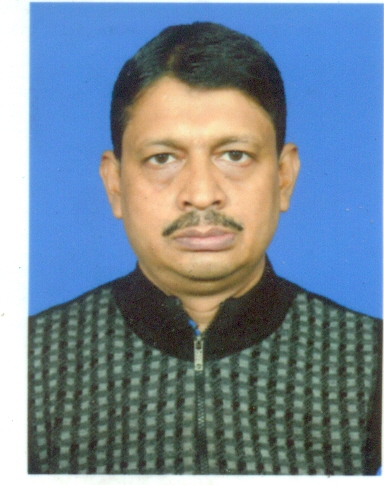Debdash Sharma
Office Staff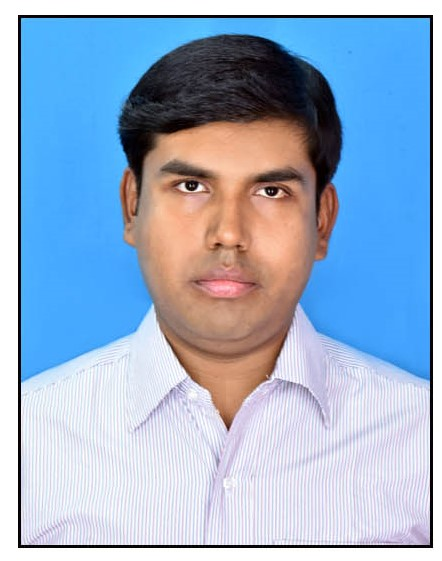Somnath Saha
Office Staff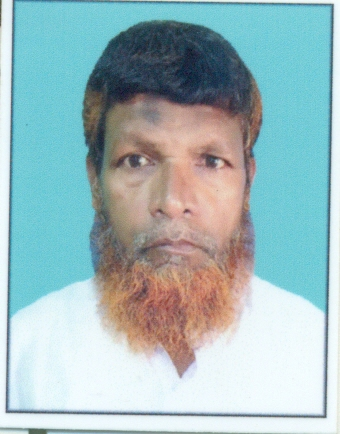Hafizul Haque
Peon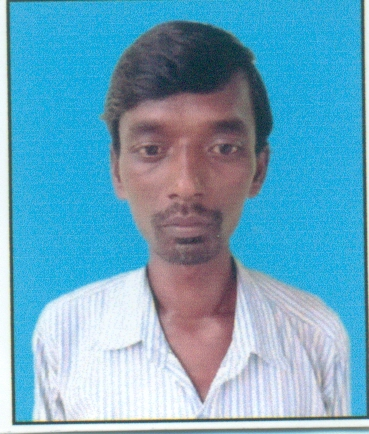Suniram Marddi
Peon
Network Support TeamRupam Das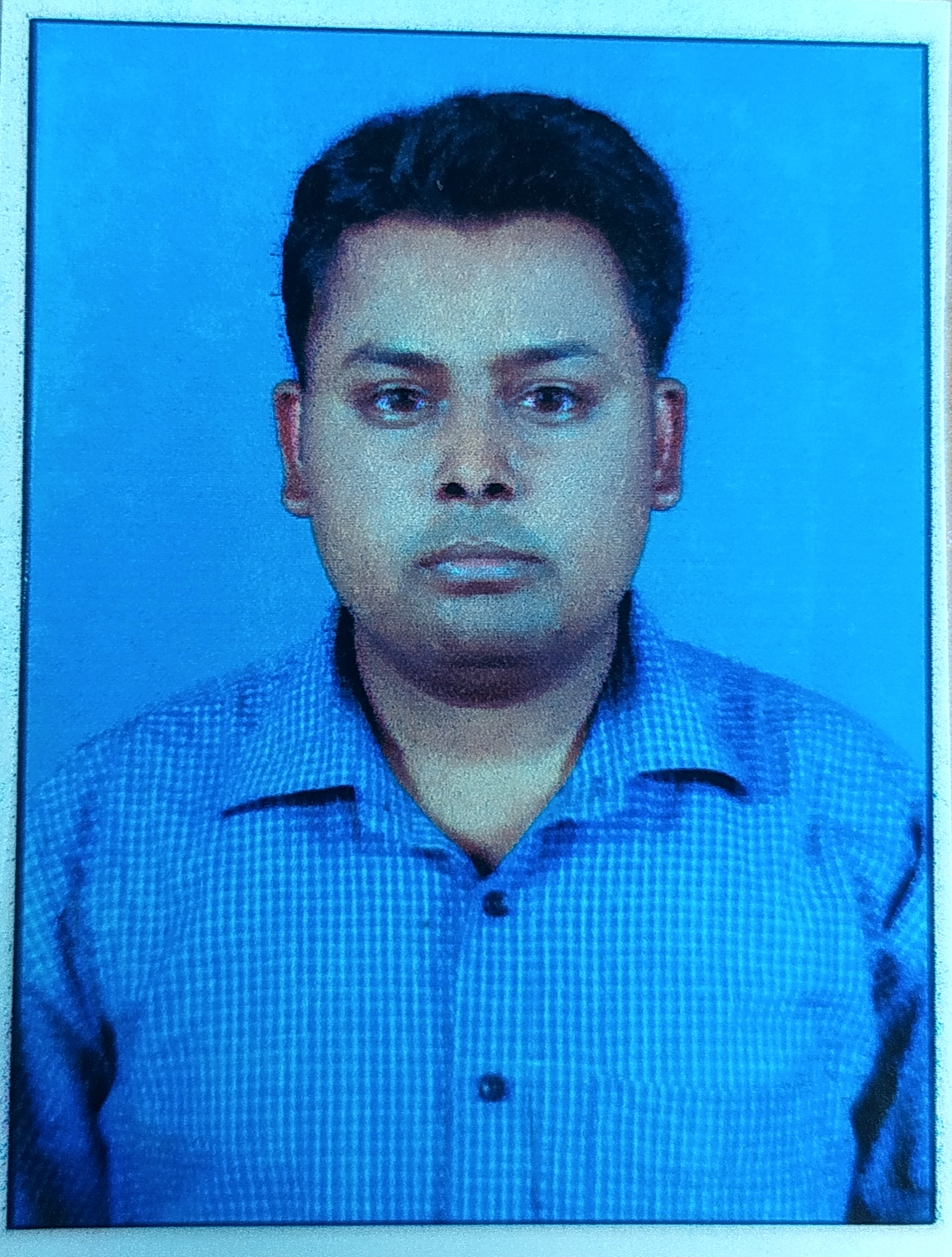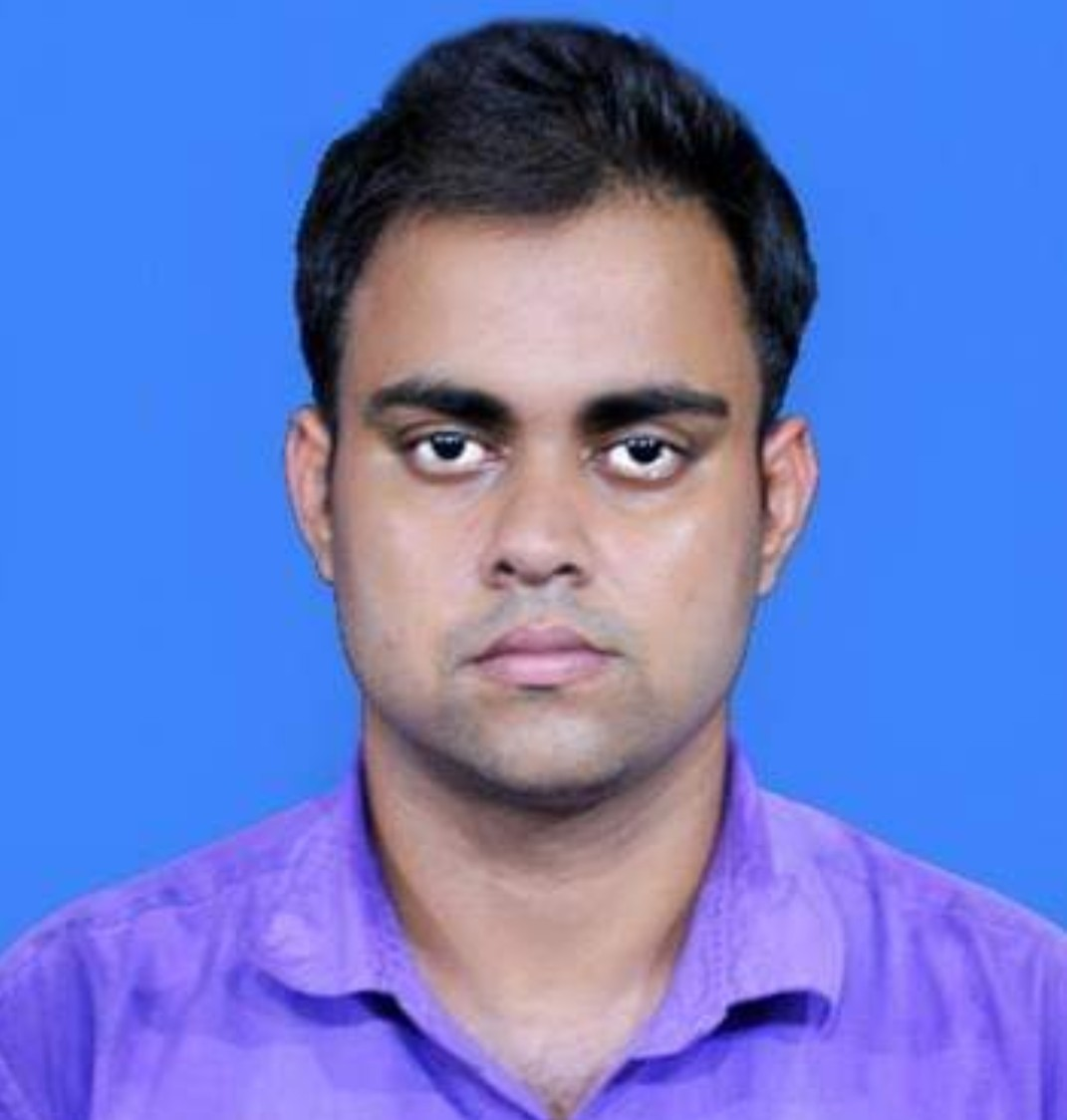Subrata Mukherjee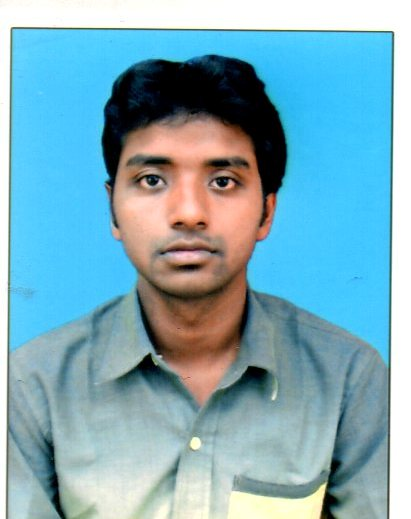Supriyo Roy
Server Support Team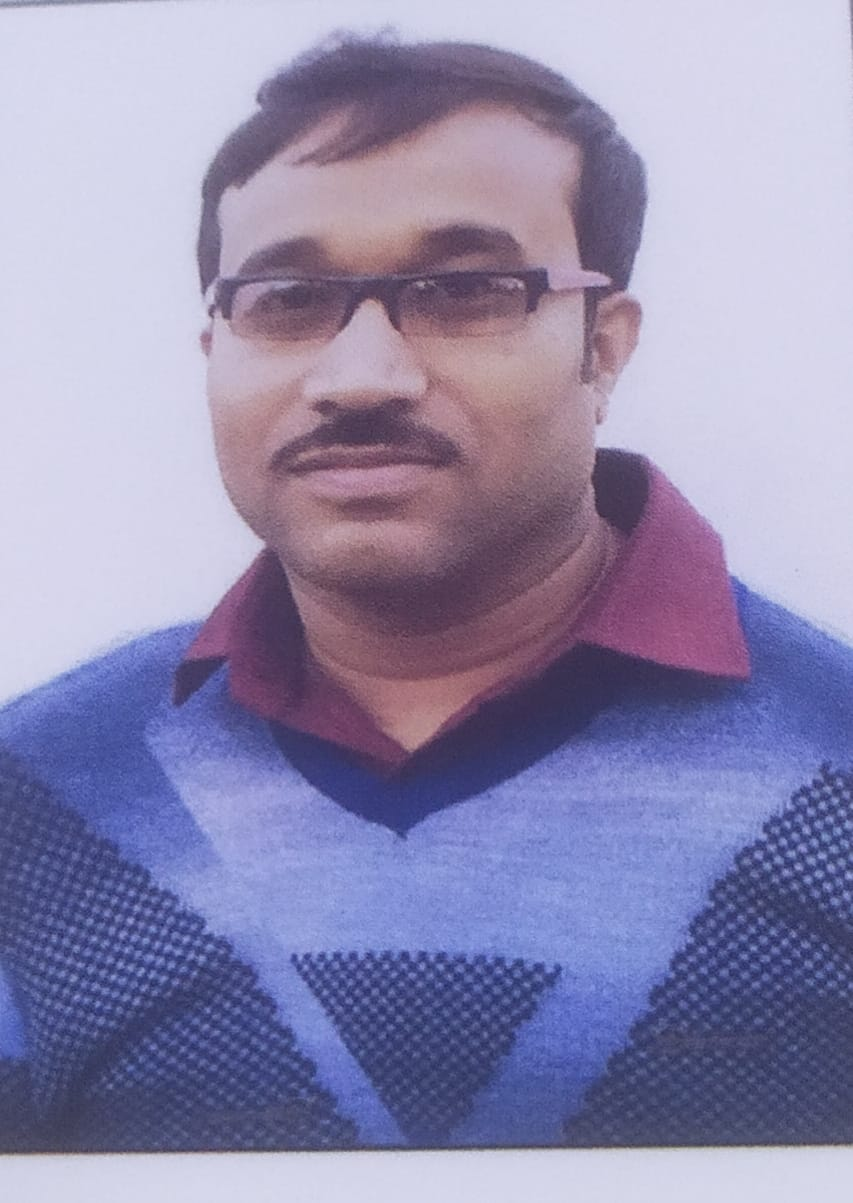Asit Kumar SahaHasibul MondalVivek Kumar Rai